NEET  >  NEET Previous Year Questions (2014-22): Thermodynamics

# NEET Previous Year Questions (2014-22): Thermodynamics - Notes | Study Physics Class 11 - NEET

 1 Crore+ students have signed up on EduRev. Have you?

Q.1. An ideal gas undergoes four different processes from the same initial state as shown in the figure below. Those processes are adiabatic, isothermal, isobaric and isochoric. The curve which represents the adiabatic process among 1, 2, 3 and 4 is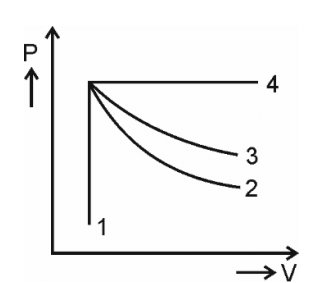(1) 2
(2) 3
(3) 4
(4) 1
Ans: 1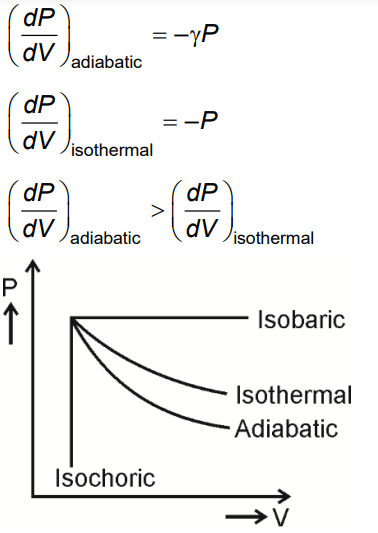Q.2. Which one among the following is the correct option for right relationship between Cp and CV for one mole of ideal gas ?      
(a) CP = RCV
(b) CP  = RCV
(c) CP + CV = R
(d) CP - CV = R
Ans:
d

Hint: CP - CV is equal to gas constant
The relation between Cp and CV for an ideal gas is as follows:
CP - CV = R
Here, CV is heat capacity at constant volume and CP is heat capacity at constant pressure.

Q.2. For irreversible expansion of an ideal gas under isothermal condition, the correct option is :     
(a) ΔU = 0, Δ Stotal ≠ 0
(b) ∆U ≠ 0, ∆ Stotal = 0
(c) ∆U = 0, ∆ Stotal = 0
(d) ∆U ≠ 0, ∆ Stotal ≠ 0
Ans:
a

Hint: ∆U = nCV∆T

In the case of isothermal process, ΔT is zero. The value of ΔU is also zero from the relation, ∆U=nCV∆T.
Thus, for reversible and irreversible expansion for an ideal gas, under isothermal conditions, ∆U = 0. But the value ∆S for irreversible expansion of an ideal gas under isothermal conditions is not equal to zero.
The total entropy change ( ∆S total) for the system and surroundings of a spontaneous process is given by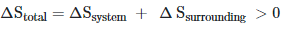Q.3. An increase in the concentration of the reactants of a reaction leads to change in :     
(a) threshold energy
(b) collision frequency
(c) activation energy
(d) heat of reaction
Ans:
b

Collision frequency, as number of molecules per unit volume increases.

(a) q < 0, ΔT = 0 and w = 0
(b) q > 0, ΔT > 0 and w > 0
(c) q = 0, ΔT = 0 and w = 0
(d) q = 0, ΔT < 0 and w > 0
Ans:
c

For an adiabatic process, q = 0
∴ w = 0
& from First law of Thermodynamics, ΔE = 0
or ΔT = 0

Q.5. For the reaction, 2Cl(g) → Cl2(g), the correct option is:      
(a) ΔrH < 0 and ΔrS > 0
(b) ΔrH < 0 and ΔrS < 0
(c) ΔrH > 0 and ΔrS > 0
(d) ΔrH > 0 and ΔrS < 0
Ans:
b

2Cl(g) → Cl2(g) + Heat
Due to bond formation stability increases which results in release of heat.
∴ ΔHr = -ve or exothermic process
ΔHr < O
& ΔS < O, because number of Cl atoms decreases in the formation of Cl2(g)

Q.6. In which of the following processes, heat is neither absorbed nor released by a system?    
(a) Isothermal
(c) Isobaric
(d) Isochoric
Ans:
b

In adiabatic process, there is no exchange of heat.

Q.7. A sample of 0.1 g of water at 100°C and normal pressure (1.013 x 105 Nm–2) requires 54 cal of heat energy to convert to steam at 100°C. If the volume of the steam produced is 167.1 cc, the change in internal energy of the sample, is:-    
(a) 104.3 J
(b) 208.7 J
(c) 42.2 J
(d) 84.5 J
Ans:
c

ΔQ = 54 cal = 54 × 4.18 joule = 225.72 joule
ΔW = P[Vsteam – Vwater] [For water 0.1 gram=0.1 cc]
= 1.013 × 105[167.1 × 10–6 – 0.1 × 10–6] joule
= 1.013 × 167 × 10–1 = 16.917 joule
By FLOT
ΔU = ΔQ – ΔW = 225.72 – 16.917
ΔU = 208.8 joule

Q.8. The volume (V) of a monatomic gas varies with its temperature (T), as shown in the graph. The ratio of work done by the gas, to the heat absorbed by it, when it undergoes a change from state A to state B, is:-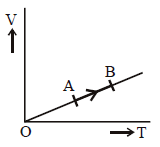(a) 2/5
(b) 2/3
(c) 1/3
(d) 2/7
Ans:
a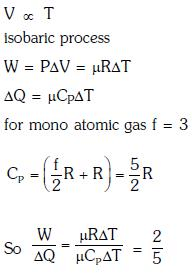Q.9. The efficiency of an ideal heat engine working between the freezing point and boiling point of water, is:-    
(a) 26.8%
(b) 20%
(c) 6.25%
(d) 12.5%
Ans:
a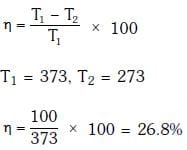Q.10. Thermodynamic processes are indicated in the following diagram: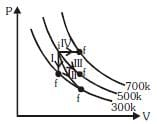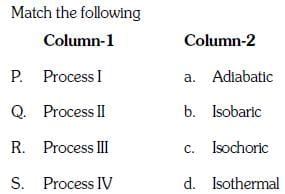(a) P → c, Q → a, R → d, S → b
(b) P → c, Q → d, R → b, S → a
(c) P → d, Q → b, R → a, S → c
(d) P → a, Q → c, R → d, S → b
Ans:
a

Process (1) → volume constant → Isochroic
Process (3) → Temperature constant → Isothermal
Process (4) → Pressure constant → Isobaric

Q.11. A carnot engine having an efficiency of 1/2 as heat engine, is used as a refrigerator. If the work done on the system is 10 J, the amount of energy absorbed from the reservoir at lower temperature is:-    
(a) 90 J
(b) 99 J
(c) 100 J
(d) 1 J
Ans:
a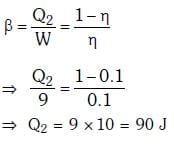Q.12. A refrigerator works between 40 celcius and 300 celcius. It is required to remove 600 calories of heat every second in order to keep the temperature of the refrigerated space constant. The power required is :(Take 1 cal = 4.2 Joules)    
(a) 2365W
(b) 2.365W
(c) 23.65W
(d) 236.5W
Ans:
d

Given,
Temperature of the source, T = 30o C = 30 + 273  = 303 K
Temperature of sink, T2 = 4o C = 4 + 273 = 277 K
We know,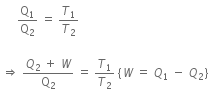where, Q2 is the amount of heat drawn from the sink at T2,
W is the work done on the working substance,
Q1 is the amount of heat rejected to source at room temperature T1.
That is,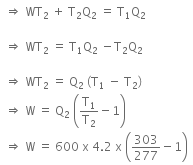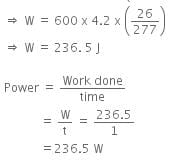Q.13. A gas is compressed isothermally to half its initial volume. The same gas is compressed separately through an adiabatic process until its volume is again reduced to half. Then    
(a) Which of the case (whether compression through isothermal or through adiabatic process) requires more work will depend upon the atomicity of the gas
(b) Compressing the gas isothermally will require more work to be done
(c) Compressing the gas through adiabatic process will require more work to be done
(d) Compressing the gas isothermally or adiabatically will require the same amount of work
Ans:
c

Directly from graph the magnitude of work done = Area under p-v plot is larger for adiabatic compression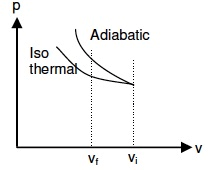Q.14. One mole of an ideal diatomic gas undergoes a transition from A to B along a path AB as shown in the figure,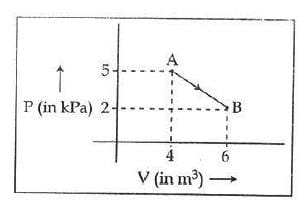The change in internal energy of the gas during the transition is :    
(a) -12 kJ
(b) 20 KJ
(c) - 20 KJ
(d) 20 J
Ans:
c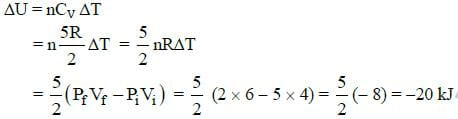Q.15. A Carnot engine, having an efficiency of η = 1/10 as heat engine, is used as a refrigerator. If the work done on the system is 10 J, the amount of energy absorbed from the reservoir at lower temperature is:    
(a) 1 J
(b) 100 J
(c) 99 J
(d) 90 J
Ans:
d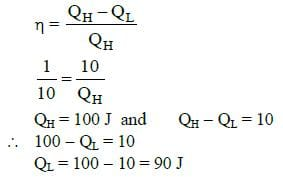Q.16. Figure below shows two paths that may be taken by a gas to go from a state A to a state C.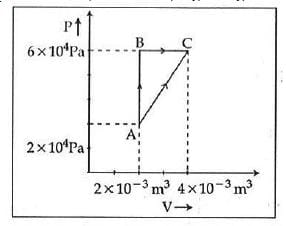In process AB, 400 J of heat is added to the system and in process BC, 100 J of heat is added to the system. The heat absorbed by the system in the process AC will be:    
(a) 300 J
(b) 380 J
(c) 500 J
(d) 460 J
Ans:
d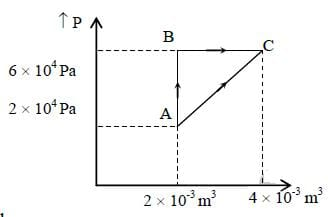See figure alongside
Process AB is isochoric so no work is done.
Heat added to be system is Q = 400 J.
Q = ΔU + ΔW
where ΔU is the change in internal energy
ΔW is the work done.
Since ΔW = 0
ΔU = Q = 400 J
Change in internal energy is 400 J.
Process BC is isobaric and the work done is given by
ΔW = P(V2 -V1) = 6 x 104(4 x 10-3 - 2 x 10-3)
= 6 x 104 x 2 x 10-3 = 120 J
Heat added to be system is Q = 100 J.
Since Q = ΔU + ΔW
∴ ΔU = Q- ΔW = (100 - 120) J = -20 J
Change in internal energy is - 20 J.Total increase in internal energy is going from state A to state C is 400 - 20 = 380 J
Work done in process AC is the area under the curve Area of the trapezium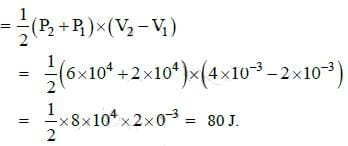Since Q = ΔU + ΔW
and ΔU the change in internal energy in process AC, we have
ΔU = 380 J and ΔW = 80 J
∴ Q = ΔU + ΔU = 380 + 80 = 460 J

Q.17. A monoatomic gas at a pressure P, having a volume V expands isothermally to a volume 2v and then adiabatically to a volume 16v. The final pressure of the gas is: (Take γ = 5/3)    
(a) P/64
(b) 16P
(c) 64P
(d) 32P
Ans:
a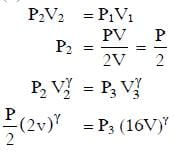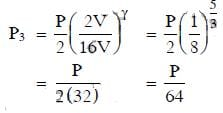Q.18. A thermodynamics system undergoes cyclic process ABCDA as shown in fig. The work done by the system in the cycle is: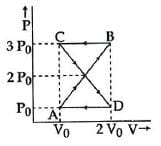(a) P0V0/2
(b) zero
(d)  2P0V0
Ans:
b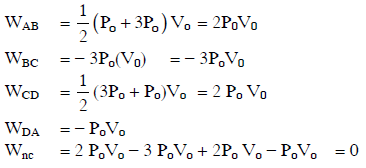The document NEET Previous Year Questions (2014-22): Thermodynamics - Notes | Study Physics Class 11 - NEET is a part of the NEET Course Physics Class 11.
All you need of NEET at this link: NEET

## Physics Class 11

127 videos|464 docs|210 tests
 Use Code STAYHOME200 and get INR 200 additional OFF

## Physics Class 11

127 videos|464 docs|210 tests

### How to Prepare for NEET

Read our guide to prepare for NEET which is created by Toppers & the best Teachers

Track your progress, build streaks, highlight & save important lessons and more!

,

,

,

,

,

,

,

,

,

,

,

,

,

,

,

,

,

,

,

,

,

;